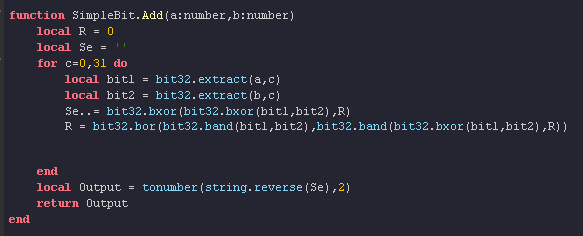# How to represent decimals in binary?

For my SimpleBit module, I have made an adder and multiplier that work 30x faster than arithmetic `*` and `+`. however, I have run into an issue, How do I represent digital numbers, and most importantly, how do I run that through the `tonumber(_,2)` to get my result?

What I was thinking was a 64 bit system,

``````00000000 00000000 00000000 00000000  00000000 00000000 00000000 00000000
``````

I assume that I could run `tonumber()` on the last 4 bytes (that represent decimal) and then do:

``````local result = tonumber(''..tonumber(PosBits,2)..'.'..tonumber(NegBits,2),2)
``````

But I am not sure if that would even work.
But what are your ideas? I am interested.

1 Like

You could use a double, they’re 64 bits: Double-precision floating-point format - Wikipedia

That’s actually what a lua number is stored as anyways. I’m assuming you’re doing this for fun thoughThis would require a lot more work than just `tonumber` though!

Decimal can be represented by the inverse of 2 to the power of whatever place you’re after.

1.5 would be 1.1b (1 * 2^0 + 1 * 2^-1 = 1 + 0.5)
5.25 would be 101.01b (1 * 2^2 + 1 * 2^0 + 1 * 2^-2 = 4 + 1 + 0.25)

You may allocate the last 4 bytes as you’ve mentioned to store strictly decimal values. However, keep in mind that IEEE 754 Floating Point is the standard format for storing decimal values now.

In terms of tonumber() and decimal places, it seems like there’s really no way to combine the two. Isolating the decimal portion then passing it through tonumber() won’t give you the right numbers. You’ll have to make your own converter or use another function/approach.

I’d also suggest taking a look at the `string.pack` functions. They’re undocumented by roblox but they’re there: Lua 5.3 Reference Manual

They let you use the characters of a string as bytes:

``````local num = 123.45

-- convert it to a string
local asAString = string.pack("d", num) -- "d" means "as double"
print(string.len(asAString)) -- 8 (like 8 bytes)

-- convert back to a number
local asANumber = string.unpack("d", asAString)
print(asANumber) -- 123.45
``````

Well, it’s all useless if it’s in Unicode! This is how you’d do it if you wanted to convert it to a binary string

``````local floor,insert = math.floor, table.insert
function basen(n,b)
n = floor(n)
local digits = "0123456789ABCDEFGHIJKLMNOPQRSTUVWXYZ"
local t = {}
local sign = ""
if n < 0 then
sign = "-"
n = -n
end
repeat
local d = (n % b) + 1
n = floor(n / b)
insert(t, 1, digits:sub(d,d))
until n == 0
return sign .. table.concat(t,"")
end

local binaryStr = string.pack("d", 124.4)
local stringStream = ""
for i=1,#binaryStr do
local c = string.byte(binaryStr:sub(i,i))
local byte = basen(c,2)
while (#byte ~= 8) do
byte = "0"..byte
end
stringStream = byte..stringStream
end
print(stringStream)
``````This is how my Bit32 adder works. I am not sure if it is compatible with that.

The list of bit32 operations for the adder is just this circuit broken down,
Which makes the adder extremely fast